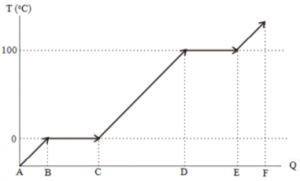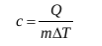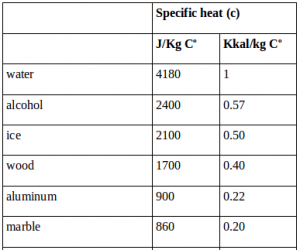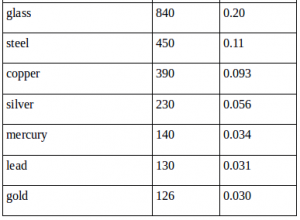# Latent heat, specific heat and heat capacity

If you put a mercury thermometer into a container containing a mixture of cold water and ice, you will observe after moving downwards, the surface of the mercury stops moving. Mercury’s surface stops moving after reaching the temperature of the freezing point of the water (0 oC). If the container is open, heat flows from the air that has the high temperature to the mixture of ice and cold water. The addition of heat from the air causes the ice to melt. As long as ice melts, does the temperature of the mixture of the ice and cold water change? As long as ice melts, the temperature of the mixture of water and ice does not change.

If a mixture of ice and water is heated using fire or an electric heater, for example, all ice turns into water. If it continues to be heated, the temperature of the water rises to 100 oC. At 100 oC, the water starts to evaporate. If the water continues to be heated, the faster the water turns into steam, while the water temperature does not change or remain 100 oC. This is the temperature of the boiling point of water, the highest temperature that can be reached by water.Graph of the relation of heat with the temperature changes and the changes phase of water

AB = Additional heat increases the ice temperature to 0 oC

BC = Additional heat melts ice into water

CD = Additional heat increases the water temperature from 0 oC to 100 oC

DE = Additional heat evaporates water

EF = Additional heat increases the temperature of the steam

The graph above shows the process of temperature changes and the changes in the water phase as long as the water absorbs heat (at one atmospheric air pressure). If the water releases heat, the process that occurs is the opposite of the process above. The addition of heat from B-C does not cause changes in water temperature, but only melts ice into water.

The addition of heat from D-E does not cause changes in water temperature, but only converts water to steam. Water is just one example. All objects will undergo a process of change in phase and temperature changes, as experienced by water if the object absorbs heat. The difference lies in the freezing point and boiling point. Every object has a different freezing point and boiling point. If objects release heat, the process that occurs is the opposite of the process above.

1. The heat of fusion (LF)

The heat of fusion is the amount of heat absorbed by 1 kg of objects to change its phase from solid to liquid, or heat released by 1 kg of objects to change its phase from liquid to solid. The formula for determining the amount of heat absorbed (or released) to change the phase of an object from solid to liquid (or liquid to solid):

Q = m LF

Description: Q = heat absorbed or released, m = mass of the object, LF = heat of fusion

2. The heat of vaporization (LV)

The heat of vaporization is the amount of heat absorbed by 1 kg of objects to change its phase from liquid to become gas, or heat released by 1 kg of objects to change its phase from gas to liquid. The formula for determining the amount of heat absorbed (or released) to convert an object from liquid to gas (or gas to liquid):

Q = m LV

Description: Q = heat absorbed or released, m = mass of object, LV = heat of vaporization

The heat of fusion and Heat of vaporization are referred to as the latent heat.

Specific heat (c)

Specific heat (c) = the amount of heat (Q) needed to raise the temperature (T) of one unit of mass (m) of objects by one degree. The equation of the specific heat :The International System Unit of the specific heat is J/Kg K.

The specific heat of an object changes with temperature. If the temperature change is not too large, the heat can be considered constant.

The following is the heat of several objects at a pressure of 1 atm and a temperature of 20 oC (obtained through experiments).Heat capacity (C)

Heat capacity (C) = the amount of heat needed to raise the temperature of all objects by one degree. The equation of the heat capacity:

C = m c

Description: C = heat capacity, m = mass, c = the specific heat of the object.